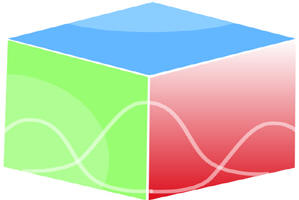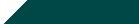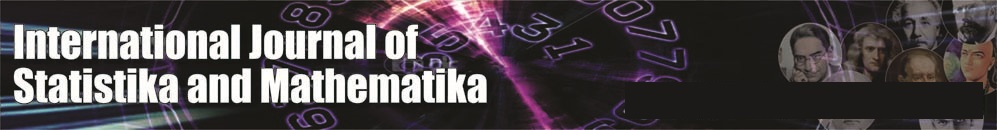``Home| Journals | Statistics Online Expert | About Us | Contact UsUntitled Document

[

Benefit-function of two-identical cold standby system subject to failure due to greenhouse effect and failure due to thermal radiation

Ashok Kumar Saini

Associate Professor, Department of Mathematics, B. L. J. S. College, Tosham, Bhiwani, Haryana, INDIA.

Email: [email protected]

Research Article

Keywords: Cold Standby, FGHE - failure due to Greenhouse effect, FTR - failure due to Thermal radiation, first come first serve, MTSF, Availability, Busy period, Benefit -Function.

INTRODUCTION

Assumptions

1. F1(t) and F2(t) are general failure time distributions due to Thermal radiation and Greenhouse effect. The repair is of two types -Type -I, Type-II with repair time distributions as G1(t) and G2(t) respectively.
2. The Thermal radiation is non-instantaneous and it cannot come simultaneously in both the units.
3. Whenever the thermal radiation occur within specified limit of the unit, it works as normal as before. But as soon as there occur Thermal radiation of magnitude beyond specified limit of the unit the operation of the unit stops automatically.
4. The repair starts immediately after the Thermal radiation of beyond specified limit of the unit are over and works on the principle of first fail first repaired basis.
5. The repair facility does no damage to the units and after repair units are as good as new.
6. The switches are perfect and instantaneous.
7. All random variables are mutually independent.
8. When both the units fail, we give priority to operative unit for repair.
9. FTR - Failure due to thermal radiation when it is beyond specified limit.
10. Repairs are perfect and failure of a unit is detected immediately and perfectly.
11. The system is down when both the units are non-operative.

SYMBOLS FOR STATES OF THE SYSTEM

F1(t) and F2(t) the failure time distribution due to Thermal radiation and Greenhouse effect respectively

G1(t), G2(t) – repair time distribution Type -I, Type-II due to Thermal radiation and Greenhouse effect respectively

Superscripts: O, CS, FGHE, FTR

Operative, Cold Standby, failure due to greenhouse effect, Failure due to thermal radiation respectively

Subscripts: ntrf, trf, ghe, ur, wr, uR

No Thermal radiation failure, Thermal radiation failure, green house effect, under repair, waiting for repair, under repair continued from previous state respectively

Up states: 0, 1, 2;

Down states: 3, 4

Regeneration point: 0, 1, 2

Notations

Mi(t) System having started from state I is up at time t without visiting any other regenerative state

Ai (t) state is up state as instant t

Ri (t) System having started from state I is busy for repair at time t without visiting any other regenerative state.

Bi (t) the server is busy for repair at time t.

Hi(t) Expected number of visits by the server for repairing given that the system initially starts from regenerative state i

States of the System

0(Ontrf, CSntrf)

One unit is operative and the other unit is cold standby and there is no Thermal radiation in both the units.

1(SOFTR trf, ur, Ontrf)

The operating unit fails due to Thermal radiation and is under repair immediately of very costly Type- I and standby unit starts operating with no Thermal radiation.

2(FGHE ntrf, ghe, ur, Ontrf)

The operative unit fails due to FGHE resulting from Greenhouse effect and undergoes repair of type II and the standby unit becomes operative with no thermal radiation.

3(FTRtrf,uR, FGHE ntrf, ghe, wr)

The first unit fails due to Thermal radiation and under very costly Type-I repair is continued from state 1 and the other unit fails due to FGHE resulting from Greenhouse effect and is waiting for repair of Type -II.

4(FTR trf, uR, FTRtrf,wr)

The one unit fails due to Thermal radiation is continues under repair of very costly Type - I from state 1 and the other unit also fails due to Thermal radiation is waiting for repair of very costly Type- I.

5(FGHE ntrf, ghe, uR, FTR trf, wr)

The operating unit fails due to Greenhouse effect (FGHE mode) and under repair of Type - II continues from the state 2 and the other unit fails due to Thermal radiation is waiting for repair of very costly Type- I.

6(FGHE ntrf,ghe,uR, FGHE ntrf,ghe,wr)

The operative unit fails due to FGHE resulting from Greenhouse effect and under repair continues from state 2 of Type –II and the other unit is also failed due to FGHE resulting from Greenhouse effect and is waiting for repair of Type-II and there is no Thermal radiation.

Figure 1: The State Transition Diagram

TRANSITION PROBABILITIES

Simple probabilistic considerations yield the following expressions:

p01 = , p02 =

p10 = , p13 =p11(3)= p11(4)=

p25 = p22(5)= p22(6) =

Clearly

p01 + p02 = 1,

p10 + p13 =(p11(3) ) + p14 = ( p11(4)) = 1,

p20 + p25 = (p22(5)) + p26 =(p22(6)) = 1                                                                                                                                             (1)

And mean sojourn time is

µ0 = E(T) =                                                                                                                                                            (2)

Mean Time To System Failure

Ø0(t) = Q01(t)[s] Ø1(t) + Q02(t)[s] Ø2(t)

Ø1(t) = Q10 (t)[s] Ø0(t) + Q13(t) + Q14(t)

Ø2(t) = Q20 (t)[s] Ø0(t) + Q25(t) + Q26(t)                                                                                                                                    (3-5)

We can regard the failed state as absorbing

Taking Laplace-Stiljes transform of eq. (3-5) and solving for

ø0*(s)  = N1(s) / D1(s)                                                                                                                                                                       (6)

Where

N1(s) = Q01*[Q13 * (s) + Q14 * (s) ] + Q02*[ Q25 * (s) + Q26 * (s) ]

D1(s) = 1 - Q01* Q10* - Q02* Q20*

Making use of relations (1) and (2) it can be shown that ø0*(0)  =1, which implies that ø0*(t)  is a proper distribution.

MTSF = E[T] =    (s)              = (D1(0) - N1(0)) / D1 (0)

s=0

= ( +p01  + p02 ) / (1 - p01 p10 - p02 p20 )

Where

= 1 + 2, 1= 0 + 3 + 4

+ +

AVAILABILITY ANALYSIS

Let Mi(t) be the probability of the system having started from state I is up at time t without making any other regenerative state. By probabilistic arguments, we have

The value of M0(t), M1(t), M2(t) can be found easily.

The point wise availability Ai(t) have the following recursive relations

A0(t) = M0(t) + q01(t)[c]A1(t) + q02(t)[c]A2(t)

A1(t) = M1(t) + q10(t)[c]A0(t) + q11(3)(t)[c]A1(t)+ q11(4)(t)[c]A1(t),

A2(t) = M2(t) + q20(t)[c]A0(t) + [q22(5)(t)[c]+ q22(6)(t)] [c]A2(t)                                                                                          (7-9)

Taking Laplace Transform of eq. (7-9) and solving for

= N2(s) / D2(s)                                                                                                                                                           (10)

Where

N2(s) =  0(s)(1 -  11(3)(s) -  11(4)(s)) (1-  22(5)(s)-  22(6)(s)) +  (s) 1(s)

[ 1-  22(5)(s)-  22(6)(s)] + 02(s)  2(s)(1- 11(3)(s) - 11(4)(s))

D2(s) = (1 -  11(3)(s)-  11(4)(s)) { 1 -  22(5)(s) - 22(6)(s) )[1-( 01(s)  10 (s))(1-

11(3)(s)-  11(4)(s))]

A0 =  =  =

Using L’ Hospitals rule, we get

A0 =  =                                                                                                                                      (11)

The expected up time of the system in (0,t] is

(t) =  So that                                                                                                    (12)

The expected down time of the system in (0, t] is

(t) = t- (t) So that                                                                                                      (13)

The expected busy period of the server when there is FGHE-failure resulting from Greenhouse effect in (0, t]

R0(t) = q01(t)[c]R1(t) + q02(t)[c]R 2(t)

R1(t) = S1(t) + q01(t)[c]R1 (t) + [q11(3)(t) + q11(4)(t)[c]R1(t),

R2(t) = q20(t)[c]R0(t) + [q22(6)(t)+q22(5)(t)][c]R2(t)                                                                                                                  (14-16)

Taking Laplace Transform of eq. (14-16) and solving for

= N3(s) / D3(s)                                                                                                                                                            (17)

Where

N 3(s) = 01(s)  1(s) and

D 3(s)= (1 -  11(3)(s)-  11(4)(s)) – 01(s) is already defined.

In the long run, R0 =                                                                                                                                                      (18)

The expected period of the system under FGHE-failure resulting from Green House effect in (0, t] is

(t) =  So that

The expected busy period of the server when there is Thermal radiation in (0, t]

B0(t) = q01(t)[c]B1(t) + q02(t)[c]B2(t)

B1(t) = q01(t)[c]B1(t) + [q11(3)(t)+ q11(4)(t)] [c]B1(t),

B2(t) = T2(t) + q02(t)[c] B2(t) + [q22(5)(t)+ q22(6)(t)] [c]B2(t)

T2(t) = e- λ1t G2(t)                                                                                                                                                                                               (19- 21)

Taking Laplace Transform of eq. (19-21) and solving for

= N4(s) / D2(s)                                                                                                                                                           (22)

Where

N4(s) = 02(s)  2(s))

In steady state, B0 =                                                                                                                                                      (23)

The expected busy period of the server for repair in (0, t] is

(t) =  So that                                                                                                                 (24)

The expected number of visits by the repairman for repairing the identical units in (0, t]

H0(t) = Q01(t)[s][1+ H1(t)] + Q02(t)[s][1+ H2(t)]

H1(t) = Q10(t)[s]H0(t)] + [Q11(3)(t)+ Q11(4)(t)] [s]H1(t),

H2(t) = Q20(t)[s]H0(t) + [Q22(5)(t) +Q22(6)(t)] [c]H2(t)                                                                                                             (25-27)

Taking Laplace Transform of eq. (25-27) and solving for

= N6(s) / D3(s)                                                                                                                                                           (28)

In the long run, H0 =                                                                                                                                                      (29)

BENEFIT- FUNCTION ANALYSIS

The Benefit-Function analysis of the system considering mean up-time, expected busy period of the system under Thermal radiation when the units stops automatically, expected busy period of the server for repair of unit under Green House effect, expected number of visits by the repairman for unit failure.

The expected total Benefit-Function incurred in (0, t] is

C (t) = Expected total revenue in (0, t]

• expected total repair cost in (0,t] due to Thermal radiation failure
• expected total repair cost due to FGHE: failure resulting from Green house effect for repairing the units in (0,t ]
• expected busy period of the system under Thermal radiation when the units automatically stop in (0, t]
• expected number of visits by the repairman for repairing of identical the units in (0, t]

The expected total cost per unit time in steady state is

C =  =

= K1A0 - K 2R0 - K 3B0 - K 4H0

Where

K1: revenue per unit up-time,

K2: cost per unit time for which the system is under repair of type- I

K3: cost per unit time for which the system is under repair of type-II

K4: cost per visit by the repairman for units repair.

CONCLUSION

After studying the system, we have analyzed graphically that when the failure rate due to green house effect and failure rate due to Thermal radiation increases, the MTSF and steady state availability decreases and the Benefit-function decreased as the failure increases.

REFERENCES

1. Dhillon, B.S. and Natesen, J, Stochastic Anaysis of outdoor Power Systems in fluctuating environment, Microelectron. Reliab.. 1983; 23, 867-881.
2. Kan, Cheng, Reliability analysis of a system in a randomly changing environment, Acta Math. Appl. Sin. 1985, 2, pp.219-228.
3. Cao, Jinhua, Stochatic Behaviour of a Man Machine System operating under changing environment subject to a Markov Process with two states, Microelectron. Reliab., 1989; 28, pp. 373-378.
4. Barlow, R.E. and Proschan, F., Mathematical theory of Reliability, 1965; John Wiley, New York.
5. Gnedanke, B.V., Belyayar, Yu.K. and Soloyer, A.D., Mathematical Methods of Relability Theory, 1969 ; Academic Press, New York.